# NCERT Exemplars - Semiconductor Electronics (Part - 1) Notes | EduRev

## JEE : NCERT Exemplars - Semiconductor Electronics (Part - 1) Notes | EduRev

The document NCERT Exemplars - Semiconductor Electronics (Part - 1) Notes | EduRev is a part of the JEE Course NEET Revision Notes.
All you need of JEE at this link: JEE

MULTIPLE CHOICE QUESTIONS I

Q.1. The conductivity of a semiconductor increases with increase in temperature because
(a) Number density of free current carriers increases
(b) Relaxation time increases
(c) Both number density of carriers and relaxation time increase
(d) Number density of current carriers increases, relaxation time decreases but effect of decrease in relaxation time is much less than increase in number density
Ans. (d)
Solution.
Key concept
Conductivity of Semiconductor:
(1) In intrinsic semiconductors ne = nh. Both electron and holes contributes in current conduction.
(2) When some potential difference is applied across a piece of intrinsic semiconductor current flows in it due to both electron and holes, i.e. i = ie  + i⇒ i = eA[nev+ nhvh]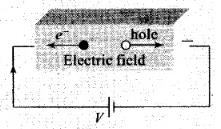(3) As we know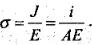Hence conductivity of semiconductor is σ = e[neμ+ nhμh]; where ve = drift velocity of electron, vh = drift velocity of holes, E= Applied electric field,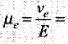mobility of electron and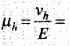mobility of holes
(4) Motion of electrons in the conduction band and of holes in the valence band under the action o f electric field is shown below: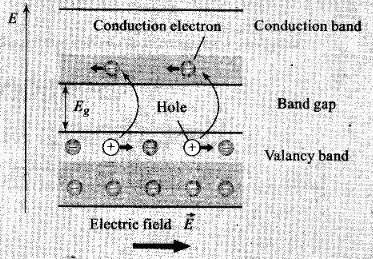(5) At absolute zero temperature (0 K)conduction band of semiconductor is completely empty, i.e., σ= 0. Hence the semiconductor behaves as an insulator.
We know that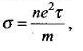So, σ ∝ nτ
where, n = number density and τ = relaxation time
In semiconductors conductivity increases with increase in temperature, because the number density of current carries increases, relaxation time decreases but effect of decrease in relaxation is much less than increase in number density.

Q.2. In Figure, Vo is the potential barrier across a p-n junction, when no battery is connected across the junction
(a) 1 and 3 both correspond to forward bias of junction
(b) 3 corresponds to forward bias of junction and 1 corresponds to reverse bias of junction (c) 1 corresponds to forward bias and 3 corresponds to reverse bias of junction.
(d) 3 and 1 both correspond to reverse bias of junction.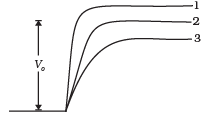Ans.
(b)
when P-n junction is in forward bias, it compresses or decreases the depletion layer so potential barrier in forward bias decreases and in reverse layer so potential barrier in forward bias decrease and in reverse bias potential barrier increases.

Q.3. In Figure assuming the diodes to be ideal,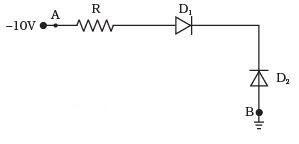(a) D1 is forward biased and D2 is reverse biased and hence current flows from A to B
(b) D2 is forward biased and D1 is reverse biased and hence no current flows from B to A and vice versa.
(c) Dand D2 are both forward biased and hence current flows from A to B.
(d) D1 and D2 are both reverse biased and hence no current flows from A to B and vice versa.
Ans.
(b)
Solution.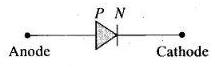A symbol of the diode is represented like this:
In this problem first we have to check the polarity of the diodes. - 10 V is the lower, voltage in the circuit. Now p-side of p-n junction D1, is connected to lower voltage and n-side of D1 to higher voltage. Thus D1 is reverse biased.
Now, let us analyse 2nd diode of the given circuit. The p-side of p-n junction D2 is at higher potential and n-side of D2 is at lower potential. Therefore D2 is forward biased.
Hence, current flows through the junction from B to A.

Q.4. A 220 V A.C. supply is connected between points A and B (in Figure). What will be the potential difference V across the capacitor?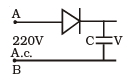(a) 220V
(b) 110V
(c) 0V
(d) 220√2 V

Ans. (d)
Solution.
Key concept: Half wave rectifier: When the P-N junction diode rectifies half of the ac wave, it is called half wave rectifier.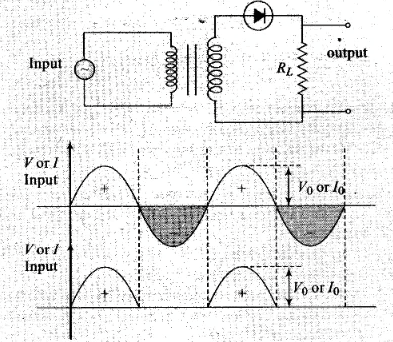(i) During positive half cycle,
Diode → forward biased
Output signal → obtained
(ii) During negative half cycle,
Diode → reverse biased
Output signal → not obtained
(iii) Output voltage is obtained across the load resistance RL. It is not constant but pulsating (mixture of ac and dc) in nature.
(iv) Average output in one cycle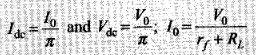(rf = forward biased resistance)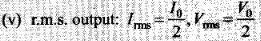As p-n junction diode will conduct during positive half cycle only, during negative half cycle diode is reverse biased. During this diode will not give any output. So, potential difference across capacitor C - peak voltage of the given AC voltage
= V0 = Vrms√2 = 220√2 V

Q.5. Hole is
(a) An anti-particle of electron
(b) A vacancy created when an electron leaves a covalent bond
(c) Absence of free electrons
(d) An artifically created particle

Ans. (b)
Solution.
Concept of holes in the semiconductor:

1. When an electron is removed from a covalent bond, it leaves a vacancy behind. An electron from a neighbouring atom can move into this vacancy, leaving the neighbour with a vacancy. In this way the vacancy formed is called a hole (or cotter), and can travel through the material and serve as an additional current carriers.
2. A hole is considered as a seat of positive charge, having magnitude of charge equal to that of an electron.
3. Holes acts as a virtual charge, although there is no physical charge on it.
4. Effective mass of hole is more than an electron.
5. Mobility of hole is less than an electron.

Q.6.The output of the given circuit in Figure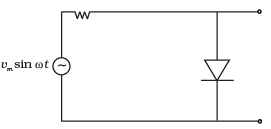(a) Would be zero at all times
(b) Would be like a half wave rectifier with positive cycles in output
(c) Would be like a half wave rectifier with negative cycles in output
(d) Would be like that of a full wave rectifier
Ans.
(c)
Solution.
When positive cycle is at A, diode will be in forward bias and resistance due to diode is approximately zero so potential across diode will be about zero.
Similarly, when there is negative half cycle at A, diode will be in reverse bias and resistance will be maximum so potential difference across diode is Vm sin ωt with negative at A.
So we get only negative output at A so it behaves like a half wave rectifier with negative cycle at A in output, verifies the answer (c).

Q. 7. In the circuit shown in Figure, if the diode forward voltage drop is 0.3 V, the voltage difference between A and B is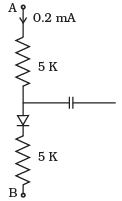(a) 1.3 V
(b) 2.3 V
(c) 0
(d) 0.5 V
Ans.
(b)
Solution.
In the middle right of the circuit the capacitor behaves like an open circuit for d.c 0.2 mA current so current will flow from A to B only. Let potential across A and B is V, so by Kirchhoff’s loop law
VAB = (5000×0.2×10-3) +0.3+5000×0.2×10-3
VAB = 1V+0.3V+ 1V
VAB = 2.3 V

Q.8. Truth table for the given circuit (Figure) is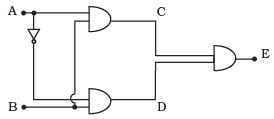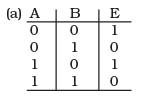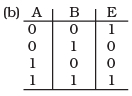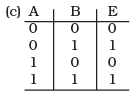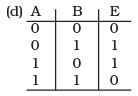Ans.
(c)
Solution.
In this problem the input C of OR gate and which is an output of AND gate. So, " equals A AND B" or C=A • B and "D equals Not A AND B" or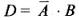and “E equals C AND D" or E = C + D = (A . B) + (A . B)
Now we can generate the truth table of this arrangement of gates can be given by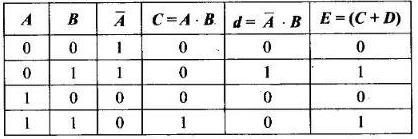MULTIPLE CHOICE QUESTIONS II

Q.9. When an electric field is applied across a semiconductor
(a) Electrons move from lower energy level to higher energy level in the conduction band
(b) Electrons move from higher energy level to lower energy level in the conduction band

(c) Holes in the valence band move from higher energy level to lower energy level
(d) Holes in the valence band move from lower energy level to higher energy level
Ans.
(a,c)
Solution.
In valence band electrons are not capable of gaining energy from external electric field. While in conduction band the electrons can gain energy from external electric field.
When electric field is applied across a semiconductor, the electrons in the conduction band (which is partially filled with electrons) get accelerated and acquire energy. They move from lower energy level to higher energy level. While the holes in valence band move from higher energy level to lower energy level, where they will be having more energy.

Q.10. Consider an npn transistor with its base-emitter junction forward biased and collector base junction reverse biased. Which of the following statements are true?.
(a) Electrons crossover from emitter to collector
(b) Holes move from base to collector
(c) Electrons move from emitter to base
(d) Electrons from emitter move out of base without going to the collector
Ans.
(a,c)
Solution.
Electrons are repelled by forward bias from emitter to collector  5% of electrons combine with holes of base and rest 95% electrons of emitter are attracted by reverse bias of collector-base junction.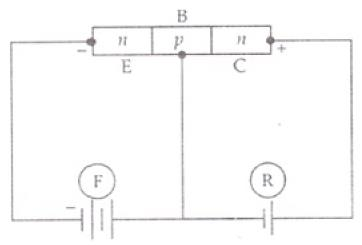So electrons move from emitter to collector through base i.e., emitter to base verifies (a) and (c).

Q.11 Figure shows the transfer characteristics of a base biased CE transistor. Which of the following statements are true?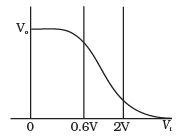(a) At Vi = 0.4 V, transistor is in active state
(b) At Vi = 1 V , it can be used as an amplifier
(c) At Vi = 0.5 V, it can be used as a switch turned off
(d) At Vi = 2.5 V, it can be used as a switch turned on
Ans.
(b,c,d)
Solution.
According to above graph transfer characteristics of a base biased common emitter transistor, we note that .
(a) when Vi= 0.4 V, output voltage remain same,there is no collection current. So, transistor circuit is not in active state.
(b) when Vi = 1 V (This is in between 0.6 V to 2 V), the transistor circuit is in active state and when input is increasing output is decreasing because when CE is used as an amplifier input and output voltages are 180° out of phase. Then it is used as an amplifier.
(c) when Vi = 0.5 V, there is no collector current. The transistor is in cut off state. The transistor circuit can be used as a switch to be turned off.
(d) when Vi = 2.5 V, the collector current becomes maximum and transistor is in a saturation state and can used as switch turned on state.

Q.12 In a npn transistor circuit, the collector  current is 10 mA. If 95 per cent of the electrons emitted reach the collector, which of the following statements are true?
(a) The emitter current will be 8 mA
(b) The emitter current will be 10.53 mA
(c) The base current will be 0.53 mA
(d) The base current will be 2 mA
Ans.
(b,c)
Solution.
According to the problem, the collector current is 95% of electrons reaching the collector after emission. And collector current, IC = 10 mA
I= emitter current
Also,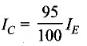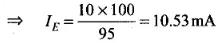Also, IB = IE - I = 10.53 - 10 = 0.53 mA

Q.13. In the depletion region of a diode
(a) there are no mobile charges
(b) equal number of holes and electrons exist, making the region neutral
(c) recombination of holes and electrons has taken place
(d) immobile charged ions exist
Ans.
(a,b,d)
Solution.
On account of difference in concentration of charge carrier in the two sections of P-N junction, the electrons from N-rcgion diffuse through the junction into P-region and the hole from P-region diffuse into N-region.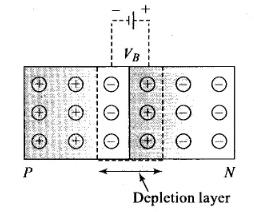Due to diffusion, neutrality of both N-and P-type semiconductor is disturbed, a layer of negative charged ions appear near the junction in the P-crystal and a layer of positive ions appears near the junction in N-crystal. This layer is called depletion layer.
The thickness of depletion layer is 1 micron = 10-6 m.
Width of depletion layer ∞  1/Dopping
Depletion is directly proportional to temperature.
Important point: The P-N junction diode is equivalent to capacitor in which the depletion layer acts as a dielectric.

Q.14. What happens during regulation action of a Zener diode?
(a) The current in and voltage across the Zenor remains fixed
(b) The current through the series Resistance (Rs) changes
(c) The Zener resistance is constant
(d) The resistance offered by the Zener changes
Ans.
(b, d)
Solution.
Symbolically zener diode represents like this:
In the forward bias, the zener diode acts as an ordinary diode. It can be used as a voltage regulator.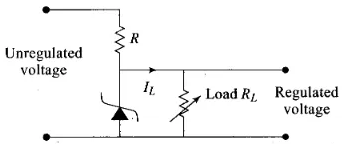Zener Diode as a voltage regulatorA zener diode when reverse biases offers constant voltage drop across in terminals as unregulated voltage is applied across circuit to regulate. Then during regulation action of a Zener diode, the current through the series resistance Rs changes and resistance offered by the Zener changes. The current through the Zener changes but the voltage across the Zener remains constant.

Q.15. To reduce the ripples in a rectifier circuit with capacitor filter
(a) RL should be increased
(b) Input frequency should be decreased
(c) Input frequency should be increased
(d) Capacitors with high capacitance should be used
Ans.
(a,c,d)
Solution.
Ripple factor may be defined as the ratio of r.m.s. value of the ripple voltage to the absolute value of the DC component of the output voltage, usually expressed as a percentage. However ripple voltage is also commonly expressed as the peak-to-peak value. Ripple factor (r) of a full wave rectifier using capacitor filter is given byWhere, L is inductance of the coil and ω is the angular frequency.
or Ripple factor  can also  be given by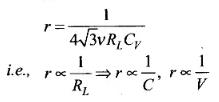Ripple factor is inversely proportional to RL, C and v.
Thus to reduce r, RL should be increased, input frequency v should be increased and capacitance C should be increased.

Q.16. The breakdown in a reverse biased p–n junction diode is more likely to occur due to (a) large velocity of the minority charge carriers if the doping concentration is small
(b) large velocity of the minority charge carriers if the doping concentration is large
(c) strong electric field in a depletion region if the doping concentration is small
(d) strong electric field in the depletion region if the doping concentration is large
Ans.
(a,d)
Solution.
Reverse biasing: Positive terminal of the battery is connected to the N-crystal and negative terminal of the battery is connected to P-crystal.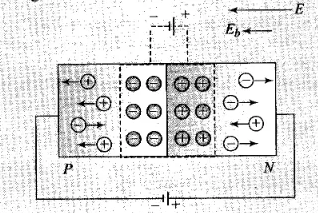(i) In reverse biasing width of depletion layer increases

(ii) In reverse biasing resistance offered RReverse = 105 Ω

(iii) Reverse bias supports the potential barrier and no current flows across the junction due to the diffusion of the majority carriers.

(A very small reverse current may exist in the circuit due to the drifting of minority carriers across the junction)

(iv) Break down voltage: Reverse voltage at which break down of semiconductor occurs. For Ge it is 25 V and for Si it is 35 V.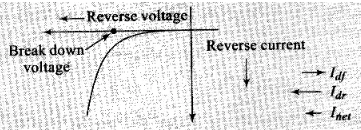So, we conclude that in reverse biasing, ionization takes place because the minority charge carriers will be accelerated due to reverse biasing and striking with atoms which in turn cause secondary electrons and thus more number of charge carriers.
When doping concentration is large, there will be a large number of ions in the depletion region, which will give rise to a strong electric field.

Offer running on EduRev: Apply code STAYHOME200 to get INR 200 off on our premium plan EduRev Infinity!

291 docs

,

,

,

,

,

,

,

,

,

,

,

,

,

,

,

,

,

,

,

,

,

;